Courses

# Solutions of Reflection of Light (Page No- 193 & 198) - Physics By Lakhmir Singh, Class 10 Class 10 Notes | EduRev

## Class 10 : Solutions of Reflection of Light (Page No- 193 & 198) - Physics By Lakhmir Singh, Class 10 Class 10 Notes | EduRev

The document Solutions of Reflection of Light (Page No- 193 & 198) - Physics By Lakhmir Singh, Class 10 Class 10 Notes | EduRev is a part of the Class 10 Course Class 10 Physics Solutions By Lakhmir Singh & Manjit Kaur.
All you need of Class 10 at this link: Class 10

Lakhmir Singh Physics Class 10 Solutions Page No:193

Question 8: Giving reasons, state the ‘signs’ (positive or negative) which can be given to the following :
(a) object distance (u) for a concave mirror or convex mirror
(b) image distances (v) for a concave mirror
(c) image distances (v) for a convex mirror

Solution : (a) Object distance (u) for a concave mirror or convex mirror is always negative because an object is always placed to the left side of the mirror and the distances towards the left of the mirror are always negative.
(b) In case of a concave mirror, if the image is formed on the left side of the mirror, then the image distance (v) will be negative and if the image is formed on the right side of the mirror, then the image distance (v) will be positive. This is because distances measured to the left of the mirror are negative and to the right of the mirror is positive.
(c) Image distances (v) for a convex mirror is always positive because the image is always formed behind the mirror.

Lakhmir Singh Physics Class 10 Solutions Page No:198

Question 1: If the magnification of a body of size 1 m is 2, what is the size of the image ?

Solution :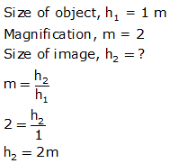Question 2: What is the position of the image when an object is placed at a distance of 20 cm from a concave mirror of focal length 20 cm ?

Solution : Object distance, u = -20 cm

Focal length. f = -20 cm (concave mirror)

Image d istance. v = ?

We know that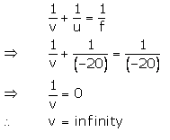Question 3: What is the nature of image formed by a concave mirror if the magnification produced by the mirror is (a) + 4, and (b) -2 ?

Solution : (a) If m = + 4. then the image is virtual and erect.
(b) If m = - 2. then the image is real and inverted.

Question 4: State the relation between object distance, image distance and focal length of a spherical mirror (concave mirror or convex mirror).

Solution :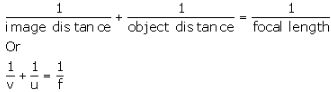v = distance of image from mirror
u = distance of object from mirror
f = focal length of mirror.

Question 5: Write the mirror formula. Give the meaning of each symbol which occurs in it.

Solution : Mirror formula is given belowv = distance of image from mirror
u = distance of object from mirror
f = focal length of mirror.

Question 6: What is the ratio of the height of an image to the height of an object known as ?

Solution : The ratio of height of an image to the height of an object is known as magnification.

Question 7: Define linear magnification produced by a mirror.

Solution : The ratio of the height of image to the height of object is known as linear magnification.

Question 8: Write down a formula for the magnification produced by a concave mirror.
(a) in terms of height of object and height of image
(b) in terms of object distance and image distance

Solution : Here = - 20 cm : f = - 10 cm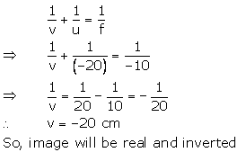Question 9: Describe the nature of image formed when the object is placed at a distance of 20 cm from a concave mirror of focal length 10 cm.

Solution : Here = - 20 cm : f = - 10 cmQuestion 10: Fill in the following blanks with suitable words :
(a) If the magnification has a plus sign, then image is…………. and……….
(b) If the magnification has a minus sign, then the image is……….. and……….

Solution : (a) virtual; erect
(b) real; inverted

Question 11: An object is placed at a distance of 10 cm from a concave mirror of focal length 20 cm.
(a) Draw a ray diagram for the formation of image.
(b) Calculate the image distance.
(c) State two characteristics of the image formed.

Solution :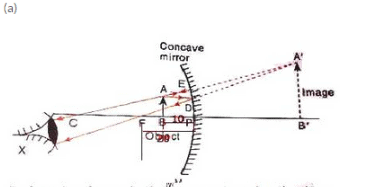fig. formation of image by the concave mirror when the object is palced between its pole and focus.

Here = - 20 cm : u = - 10 cm v = ?

We know that(c) Characteristics of image formed
(i) Image is virtual.
(ii) Image is erect.

Question 12: If an object of 10 cm height is placed at a distance of 36 cm from a concave mirror of focal length 12 cm, find the position, nature and height of the image.

Solution : h1 = 10cm. u = -36cm, f= -12cm

We know that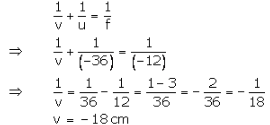∴ The position of the image is 18 cm in front of the mirror.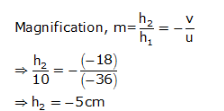The image formed is real and inverted.

Question 13: At what distance from a concave mirror of focal length 10 cm should an object 2 cm long be placed in order to get an erect image 6 cm tall ?

Solution : f=-10cm. h1 = 2cm. h2 = 6cm (erect image)
u = ?
We know that: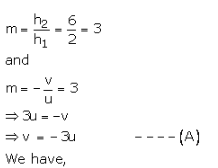The object should be placed at a distance of 6.66 cm on the left side of the mirror.

Question 14: When an object is placed at a distance of 15 cm from a concave mirror, its image is formed at 10 cm in front of the mirror. Calculate the focal length of the mirror.

Solution : u = -15 cm, v = -10cm
f = ?

We know that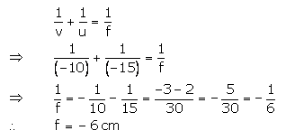Question 15: An object 3 cm high is placed at a distance of 8 cm from a concave mirror which produces a virtual image 4.5 cm high :
(i) What is the focal length of the mirror ?
(ii) What is the position of image ?
(iii) Draw a ray-diagram to show the formation of image.

Solution : h= 3cm. u = -8cm, h2 = 4.5 (virtual image)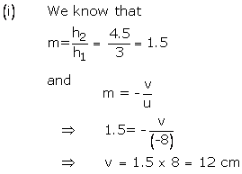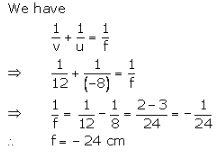(ii) v = 12cm

So, the image is formed 12 cm behind the concave mirror.

Question 16: A converging mirror forms a real image of height 4 cm of an object of height 1 cm placed 20 cm away from the mirror :
(i) Calculate the image distance.
(ii) What is the focal length of the mirror ?

Solution :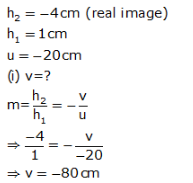Image forms in front of the concave mirror.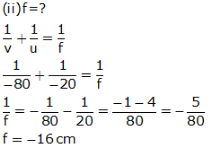Question 17: An object of size 7.0 cm is placed at 27 cm in front of a concave mirror of focal length 18 cm. At what distance from the mirror should a screen be placed so that a sharp focussed image can be obtained ? Find the size and nature of image.
[Hint. Find the value of image distance (v) first. The screen should be placed from the mirror at a distance equal to image distance].

Solution : Given : h1 = 7 cm, u = -27cm, f = -18cm,

We know that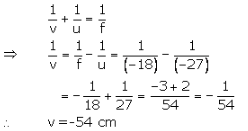The screen should be placed at a distance of 54 cm in front of the concave mirror.

And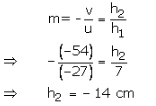Image is 14 cm in size, real and inverted.

Offer running on EduRev: Apply code STAYHOME200 to get INR 200 off on our premium plan EduRev Infinity!

94 docs

,

,

,

,

,

,

,

,

,

,

,

,

,

,

,

,

,

,

,

,

,

,

,

,

;